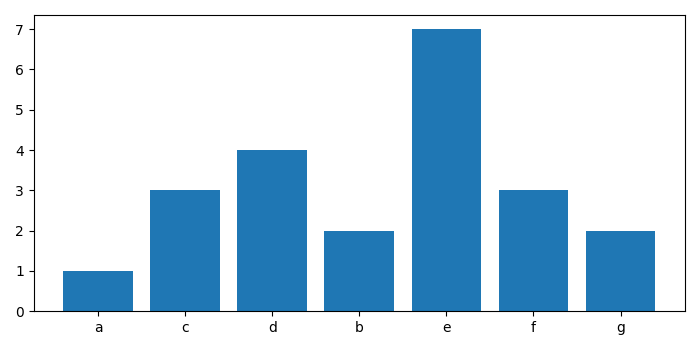# Matplotlib â€“ Make a Frequency histogram from a list with tuple elements in Python

To make a frequency histogram from a list with tuple elements in Python, we can take the following steps −

• Set the figure size and adjust the padding between and around the subplots.
• Make a list of tuples, data.
• Make lists of frequency and indices, after iterating the data.
• Make a bar plot usig bar() method.
• To display the figure, use show() method.

## Example

import matplotlib.pyplot as plt

plt.rcParams["figure.figsize"] = [7.00, 3.50]
plt.rcParams["figure.autolayout"] = True

data = [("a", 1), ("c", 3), ("d", 4), ("b", 2),
("e", 7), ("f", 3), ('g', 2)]
ind = []
fre = []
for item in data:
ind.append(item)
fre.append(item)

plt.bar(ind, fre)

plt.show()

## Output

It will produce the following output −